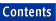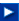Apache C++ Standard Library User's Guide

## 11.3 Example Program: Event-Driven Simulation

An extended example will now illustrate one of the more common uses of a priority_queues, which is to support the construction of a simulation model. A discrete event-driven simulation is a popular simulation technique. Objects in the simulation model objects in the real world, and are programmed to react as much as possible as the real objects would react. A priority_queue is used to store a representation of events that are waiting to happen. This queue is stored in order, based on the time the event should occur, so the smallest element will always be the next event to be modeled. As an event occurs, it can spawn other events. These subsequent events are placed into the queue as well. Execution continues until all events have been processed.

NOTE -- The complete version of the ice cream store simulation program is in icecream.cpp.

Events can be represented as subclasses of a base class, which we call event. The base class simply records the time at which the event will take place. A pure virtual function named processEvent is invoked to execute the event:

```class event {
public:
// Construct sets time of event.
event (unsigned int t) : time (t)
{ }

// Execute event by invoking this method.
virtual void processEvent () = 0;

const unsigned int time;
};
```

The simulation queue needs to maintain a collection of different types of events, sometimes called a heterogeneous collection. Each different form of event is represented by a subclass of class event, but not all events have the same exact type. For this reason the collection must store pointers to events, instead of the events themselves. Since the containers maintain pointers to values, not the values themselves, the programmer is responsible for managing the memory for the objects being manipulated.

Since comparison of pointers cannot be specialized on the basis of the pointer types, we must instead define a new comparison function for pointers to events. In the C++ Standard Library we do this by defining a new structure whose sole purpose is to define the function invocation operator()() in the appropriate fashion. Since in this particular example we want to use the priority_queue to return the smallest element each time, rather than the largest, the order of the comparison is reversed, as follows:

```struct eventComparator {
bool operator() (const event * left, const event * right) const {
return left->time > right->time;
}
};
```

NOTE -- We use a priority queue as a structure for quickly discovering the smallest element in a sequence. If, instead, your problem requires the discovery of the largest element, there are various possibilities. One is to supply the inverse operator as either a template argument or the optional comparison function argument to the constructor. If you are defining the comparison argument as a function, as in the example problem, another solution is to simply invert the comparison test.

We are now ready to define the class simulation, which provides the structure for the simulation activities. The class simulation provides two functions: the first is used to insert a new event into the queue, while the second runs the simulation. A data member is also provided to hold the current simulation time:

```class simulation {
public:
simulation () : time (0), eventQueue ()
{}
void run ();
void  scheduleEvent (event * newEvent) {
eventQueue.push (newEvent);
}
unsigned int time;
protected:
std::priority_queue<event*,
std::vector<event *, std::allocator<event*> >,
eventComparator> eventQueue;
};
```

Notice the declaration of the priority_queue used to hold the pending events. In this case we are using a vector as the underlying container, but we could just as easily have used a deque.

The heart of the simulation is the member function run(), which defines the event loop. This procedure makes use of three of the five priority_queue operations, namely top(), pop(), and empty(). It is implemented as follows:

```void simulation::run () {

while (! eventQueue.empty ()) {

event * nextEvent = eventQueue.top ();
eventQueue.pop ();
time = nextEvent->time;
nextEvent->processEvent ();
delete nextEvent;
}
}
```

### 11.3.1 Example Program: An Ice Cream Store Simulation

To illustrate the use of our simulation framework, this example program gives a simple simulation of an ice cream store. Such a simulation might be used, for example, to determine the optimal number of chairs that should be provided, based on assumptions such as the frequency with which customers arrive, the length of time they stay, and so on.

Our store simulation is based around a subclass of class simulation, defined as follows:

```class storeSimulation : public simulation {
public:
storeSimulation () : simulation (), freeChairs (35), profit (0.0)
{ }
bool canSeat (unsigned int numberOfPeople);
void order   (unsigned int numberOfScoops);
void leave   (unsigned int numberOfPeople);
// Data fields.
unsigned int freeChairs;
double       profit;
} theSimulation;
```

There are three basic activities associated with the store: arrival, ordering and eating, and leaving. This is reflected not only in the three member functions defined in the simulation class, but in three separate subclasses of event.

The member functions associated with the store simply record the activities taking place, producing a log that can later be studied to evaluate the simulation.

```bool storeSimulation::canSeat (unsigned int numberOfPeople) {

std::cout << "Time: " << time;
std::cout << " group of " << numberOfPeople << " customers arrives";

if (numberOfPeople < freeChairs) {
std::cout << " is seated\n";
freeChairs -= numberOfPeople;
return true;
}
else {
std::cout << " no room, they leave\n";
return false;
}
}

void storeSimulation::order (unsigned int numberOfScoops) {

std::cout << "Time: " << time << " serviced order for "
<< numberOfScoops << '\n';
profit += 0.35 * numberOfScoops;
}

void storeSimulation::leave (unsigned int numberOfPeople) {

std::cout << "Time: " << time << " group of size "
<< numberOfPeople << " leaves\n";
freeChairs += numberOfPeople;
}
```

As we noted already, each activity is matched by a subclass of event. Each subclass of event includes an integer data member, which represents the size of a group of customers. The arrival event occurs when a group enters. When executed, the arrival event creates and installs a new instance of the order event. The function randomInteger() is used to compute a random integer between 1 and the argument value (see Section 2.2.5).

```class arriveEvent : public event {
public:
arriveEvent (unsigned int t, unsigned int groupSize)
: event (t), size (groupSize)
{ }
virtual void processEvent ();
private:
unsigned int size;
};

void arriveEvent::processEvent () {

if (theSimulation.canSeat (size))
theSimulation.scheduleEvent
(new orderEvent (time + 1 + irand (4), size));
}
```

An order event similarly spawns a leave event:

```class orderEvent : public event {
public:
orderEvent (unsigned int t, unsigned int groupSize)
: event (t), size (groupSize)
{ }
virtual void processEvent ();
private:
unsigned int size;
};

void orderEvent::processEvent () {

// Each person orders some number of scoops.
for (unsigned int i = 0; i < size; i++)
theSimulation.order (1 + irand (4));

// Then we schedule the leave event.
theSimulation.scheduleEvent
(new leaveEvent (time + 1 + irand (10), size));
}

```

Finally, leave events free up chairs, but do not spawn any new events:

```class leaveEvent : public event
{
public:
leaveEvent (unsigned int t, unsigned int groupSize)
: event (t), size (groupSize)
{ }
virtual void processEvent ();
private:
unsigned int size;
};

void leaveEvent::processEvent () {

theSimulation.leave (size);
}
```

To run the simulation we simply create some number of initial events (say, 30 minutes worth), then invoke the run() member function:

```int main () {

std::cout << "Ice Cream Store simulation from Chapter 9\n";

// Load queue with some number of initial events.
for (unsigned t = 0; t < 20; t += irand (6)) {

std::cout << "pumping queue with event " << t << '\n';
theSimulation.scheduleEvent (new arriveEvent (t, 1 + irand (4)));
}

// Run the simulation.
theSimulation.run ();

std::cout << "Total profits " << theSimulation.profit
<< "\nEnd of ice cream store simulation\n";

return 0;
}
```